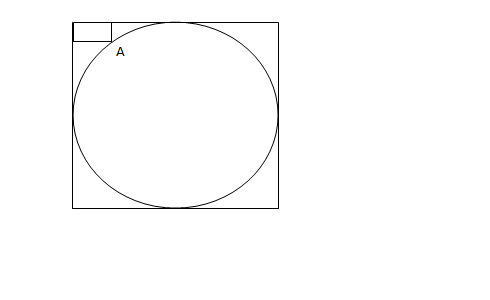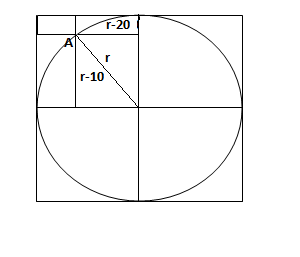Question 89

# In the figure below, the rectangle at the corner measures 10 cm × 20 cm. The corner A of the rectangle is also a point on the circumference of the circle. What is the radius of the circle in cm?SolutionAs seen in the fig. we have a right angled triangle with sides  r ,r-10 , r-20.

Using pythagoras we have $$r^2 = (r-10)^2 + (r-20)^2$$.

Solving the equation, we get r = 10 or 50.

But 10 is not possible , so r = 50.